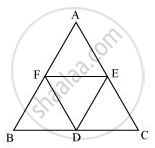Share
Notifications

View all notifications
Books Shortlist
Your shortlist is empty

# D, E and F are respectively the mid-points of the sides BC, CA and AB of a ΔABC. Show that - CBSE Class 9 - Mathematics

Login
Create free account

Forgot password?
ConceptTriangles on the Same Base and Between the Same Parallels

#### Question

D, E and F are respectively the mid-points of the sides BC, CA and AB of a ΔABC. Show that

(i) BDEF is a parallelogram.

(ii) ar (DEF) = 1/4ar (ABC)

(iii) ar (BDEF) = 1/2ar (ABC)

#### Solution(i) In ΔABC,

E and F are the mid-points of side AC and AB respectively.

Therefore, EF || BC and EF = 1/2BC (Mid-point theorem)

However, BD = 1/2BC (D is the mid-point of BC)

Therefore, BD = EF and BD || EF

Therefore, BDEF is a parallelogram.

(ii) Using the result obtained above, it can be said that quadrilaterals BDEF, DCEF, AFDE are parallelograms.

We know that diagonal of a parallelogram divides it into two triangles of equal area.

∴Area (ΔBFD) = Area (ΔDEF) (For parallelogram BD)

Area (ΔCDE) = Area (ΔDEF) (For parallelogram DCEF)

Area (ΔAFE) = Area (ΔDEF) (For parallelogram AFDE)

∴Area (ΔAFE) = Area (ΔBFD) = Area (ΔCDE) = Area (ΔDEF)

Also,

Area (ΔAFE) + Area (ΔBDF) + Area (ΔCDE) + Area (ΔDEF) = Area (ΔABC)

⇒ Area (ΔDEF) + Area (ΔDEF) + Area (ΔDEF) + Area (ΔDEF) = Area (ΔABC)

⇒ 4 Area (ΔDEF) = Area (ΔABC)

⇒ Area (ΔDEF) = 1/4Area (ΔABC)

(iii) Area (parallelogram BDEF) = Area (ΔDEF) + Area (ΔBDF)

⇒ Area (parallelogram BDEF) = Area (ΔDEF) + Area (ΔDEF)

⇒ Area (parallelogram BDEF) = 2 Area (ΔDEF)

⇒ Area (parallelogram BDEF)= 2xx1/4"Area "(ΔABC)

⇒ Area (parallelogram BDEF) = 1/2Area (ΔABC)

Is there an error in this question or solution?

#### APPEARS IN

NCERT Solution for Mathematics Class 9 (2018 to Current)
Chapter 9: Areas of Parallelograms and Triangles
Ex.9.30 | Q: 5 | Page no. 163
Solution D, E and F are respectively the mid-points of the sides BC, CA and AB of a ΔABC. Show that Concept: Triangles on the Same Base and Between the Same Parallels.
S